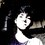# Evaluating Limit approaching Infinity.

Past sunday i gave a mathematics olympiad exam at IIT Bombay. I was asked a problem in which i had to find limit of problem :

limit x approaches infinity. $\frac{1^{3} + 2^{3} + 3^{3} + … n^{3}}{n^{4}}$

there are 2 ways of evaluating this limit.

1) by using the fact that $∑n^{3} = \frac{n^{2}(n+1)^{2}}{4}$

we get the value of limit as $\frac{1}{4}$

2) by separating the denominators and using the fact that limit of a sum is the sum of the limits.

$\frac{1^{3} + 2^{3} + 3^{3} + … n^{3}}{n^{4}}$

= $\frac{1^{3}}{n^{4}} + \frac{2^{3}}{n^{4}} + … + \frac{n^{3}}{n^{4}}$

And it turns out that the value of limit is 0.

I was confused at this while writing the exam. So I choosed $\frac{1}{4}$ by random.

And I am wondering if I did right decision or not. So I asked here. Please consider explaining me why we get two distinct answers and if my first or second approaches have any errors then please tell me. ThanksNote by Soham Zemse
7 years, 7 months ago

This discussion board is a place to discuss our Daily Challenges and the math and science related to those challenges. Explanations are more than just a solution — they should explain the steps and thinking strategies that you used to obtain the solution. Comments should further the discussion of math and science.

When posting on Brilliant:

• Use the emojis to react to an explanation, whether you're congratulating a job well done , or just really confused .
• Ask specific questions about the challenge or the steps in somebody's explanation. Well-posed questions can add a lot to the discussion, but posting "I don't understand!" doesn't help anyone.
• Try to contribute something new to the discussion, whether it is an extension, generalization or other idea related to the challenge.

MarkdownAppears as
*italics* or _italics_ italics
**bold** or __bold__ bold
- bulleted- list
• bulleted
• list
1. numbered2. list
1. numbered
2. list
Note: you must add a full line of space before and after lists for them to show up correctly
paragraph 1paragraph 2

paragraph 1

paragraph 2

[example link](https://brilliant.org)example link
> This is a quote
This is a quote
    # I indented these lines
# 4 spaces, and now they show
# up as a code block.

print "hello world"
# I indented these lines
# 4 spaces, and now they show
# up as a code block.

print "hello world"
MathAppears as
Remember to wrap math in $$ ... $$ or $ ... $ to ensure proper formatting.
2 \times 3 $2 \times 3$
2^{34} $2^{34}$
a_{i-1} $a_{i-1}$
\frac{2}{3} $\frac{2}{3}$
\sqrt{2} $\sqrt{2}$
\sum_{i=1}^3 $\sum_{i=1}^3$
\sin \theta $\sin \theta$
\boxed{123} $\boxed{123}$

Sort by:

You chose correct answer. The 2nd method is wrong. It is expressed as sum of infinite infinitesimal quantities. It is something like saying 0 multiplied by infinity.

- 7 years, 7 months ago

Cool explanation! The problem can be also evaluated using Riemann Sums which would be integration from $x=0$ to $x=1$, the function $x^{3}$.

- 7 years, 7 months ago

Exactly.

- 7 years, 7 months ago